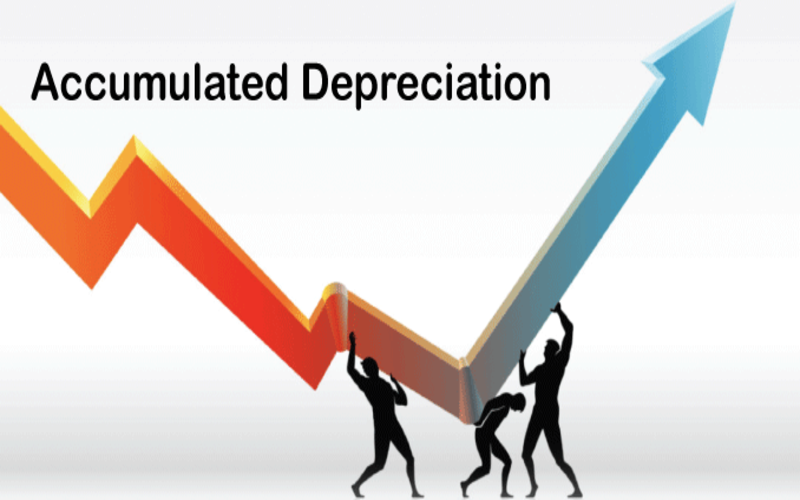Connect with us

Hi, what are you looking for?

DOGE0.070.84%SOL19.370.72%USDC1.000.01%BNB287.900.44%AVAX15.990.06%XLM0.080.37%

# Accumulated Depreciation: Everything You Need To KnowAccumulated Depreciation: Everything You Need To Know. This comprehensive guide delves into the intricate world of accumulated depreciation, shedding light on this essential accounting concept. Accumulated depreciation is crucial in financial reporting and decision-making for businesses and investors.

### What is Accumulated Depreciation?

Accumulated depreciation is an accounting method used to allocate the cost of tangible assets over their useful life. This process helps companies accurately reflect the declining value of their assets on their balance sheets. It is a key component of the depreciation expense, which impacts a company’s profitability and tax liability.

### The Importance of Accumulated Depreciation

1. Accurate Financial Reporting

Accumulated depreciation allows companies to present their financial statements more accurately. By spreading the cost of an asset over its useful life, it prevents the overstatement of asset values on the balance sheet.

2. Tax Benefits

Depreciation, including accumulated depreciation, can result in substantial business tax benefits. It lowers taxable income, reducing the income tax a company must pay.

3. Asset Replacement Planning

Understanding accumulated depreciation helps companies plan for the replacement of aging assets. Knowing how much an asset has depreciated, they can budget for future replacements more effectively.

### Calculating Accumulated Depreciation

Accumulated depreciation is calculated using a straightforward formula:

Accumulated Depreciation=Original Cost of Asset−Accumulated Depreciation to Date

### Example: Calculating Accumulated Depreciation

Let’s consider an example to illustrate this concept. Suppose a company purchases a delivery van for $30,000. Over three years, the accumulated depreciation would look like this: • Year 1:$30,000 – $10,000 =$20,000
• Year 2: $30,000 –$20,000 = $10,000 • Year 3:$30,000 – $30,000 =$0

### Impact on Financial Statements

Accumulated depreciation directly affects a company’s financial statements, primarily the balance sheet and income statement.

Balance Sheet

On the balance sheet, accumulated depreciation is subtracted from the total value of the assets to determine their net book value. This net value is a more accurate representation of the company’s assets.

Income Statement

Accumulated depreciation also impacts the income statement. The depreciation expense, which contributes to accumulated depreciation, is recognized as an expense on the income statement. This reduces the company’s reported profit, which can have tax benefits.

### Depreciation Methods

Several methods can be used to calculate depreciation, including:

1. Straight-Line Depreciation: Allocating an equal amount of depreciation expense each year.
2. Declining Balance Depreciation: Front-loading depreciation, with higher expenses in the early years.
3. Units of Production Depreciation: Depreciating assets based on their usage or production output.

The choice of method depends on various factors, including the asset’s nature and expected usage.

### Summary

• Long-term capital assets incur depreciation charges to account for the time value of money.
• The total amount of depreciation accounted for up to a certain date is the asset’s accumulated depreciation.\
• Below the line for the corresponding capital asset, a total for accumulated depreciation is shown.
• In contrast to asset accounts, which typically have debit balances, accumulated depreciation is reported as a counter asset with a credit balance.
• An asset’s carrying value equals its original purchase price less any accrued depreciation.

Accumulated depreciation is a fundamental concept in accounting and finance, enabling companies to accurately represent the value of their assets, plan for replacements, and enjoy tax benefits. Understanding how to calculate and utilize accumulated depreciation is crucial for businesses aiming to make informed financial decisions.

This guide has provided a comprehensive overview of accumulated depreciation, its importance, calculation methods, and its impact on financial statements. Armed with this knowledge, you are better equipped to navigate the world of finance and accounting.